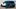Motor Trend has taken a deeper dive into the 2.28 seconds (0-60 mph) acceleration record by Tesla Model S P100D Ludicrous +, already discussed by Engineering Explained. The P100DL was especially interesting because it's a rare case of a car that can accelerate to 60 miles per hour in less time than it takes to brake back down to 0 mph.Tesla P100DL

Here's the breakdown of acceleration's stage:

• T≥ -1 minute: Ludicrous+ mode activation
• T≥ -2.00 sec: Launch mode activation
• T≥ -1.00 sec: Launch mode ready (accelerator engaged, brakes holds the car in place)
• T= -0.26 seconds: driver releases brake and car accelerates (combined output from two motors: 791 lb-ft of torque, 0.71g acceleration averaged over the first foot of travel)
• T= 0.00 sec: Timer running after 1-foot mark (5.9 mph, average 1.30 g of longitudinal acceleration)
• T= 0.40 sec: peak acceleration at 10.3 mph - 1.41 g
• T= 0.87 sec: at 30 mph - 1.14 g, distance covered: 24 feet 8 inches (1.5 car lengths)
• T= 1.30 sec: peak battery current: 1,850 amps
• T= 1.76 sec: combined motor output power peaks at 680 hp at 51.0 mph. Average acceleration at 10-50 mph is 1.14 g
• T= 2.28 sec: 60 mph speed after 120 feet 2 inches (7.4 car lengths) using 0.33 kWh of energy
• T= 10.52 sec: at 125 mph motors are spinning at 14,600 rpm (front) and 14,200 rpm (rear). Acceleration decreases to 0.22 g. Energy used - 1.53 kWh
• average deceleration while braking from 50 mph to 10 mph is 1.11 g
Source: Motor Trend